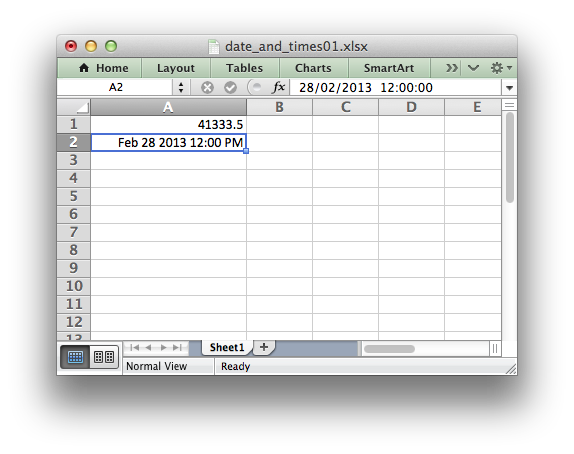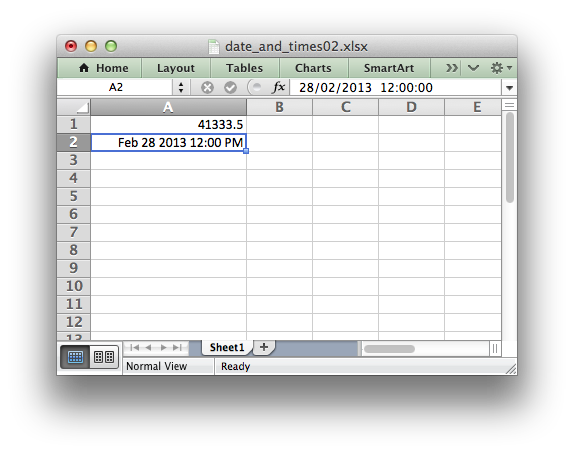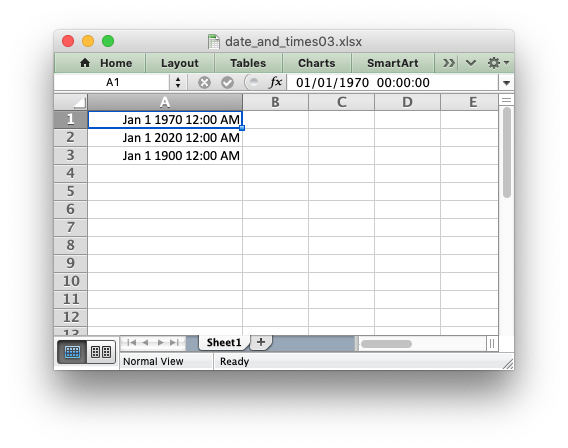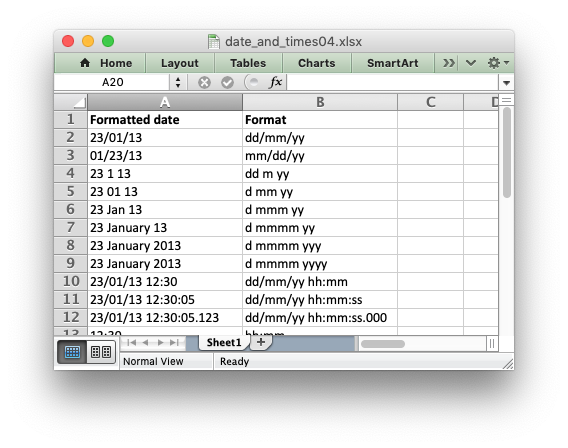libxlsxwriter
Working with Dates and Times

Dates and times in Excel are represented by real numbers. For example a date that is displayed in Excel as "Jan 1 2013 12:00 PM" is stored as the number 41275.5.

The integer part of the number stores the number of days since the epoch, which is generally 1900, and the fractional part stores the percentage of the day.

A date or time in Excel is just like any other number. To display the number as a date you must apply an Excel number format to it. Here is an example:

#include "xlsxwriter.h"
int main() {
/* A number to display as a date. */
double number = 41333.5;
/* Create a new workbook and add a worksheet. */
lxw_workbook *workbook = workbook_new("date_and_times01.xlsx");
/* Add a format with date formatting. */
format_set_num_format(format, "mmm d yyyy hh:mm AM/PM");
/* Widen the first column to make the text clearer. */
worksheet_set_column(worksheet, 0, 0, 20, NULL);
/* Write the number without formatting. */
worksheet_write_number(worksheet, 0, 0, number, NULL ); // 41333.5
/* Write the number with formatting. Note: the worksheet_write_datetime()
* or worksheet_write_unixtime() functions are preferable for writing
* dates and times. This is for demonstration purposes only.
*/
worksheet_write_number(worksheet, 1, 0, number, format); // Feb 28 2013 12:00 PM
return workbook_close(workbook);
}Some options for creating or converting dates to the correct format are shown below.

# Writing datetimes with the lxw_datetime struct

To make working with dates and times a little easier the `libxlsxwriter` library provides the lxw_datetime struct and the `worksheet_write_datetime()` function.

The members of the lxw_datetime struct and the range of their values are:

Member Value
year 1900 - 9999
month 1 - 12
day 1 - 31
hour 0 - 23
min 0 - 59
sec 0 - 59.999

Dates in Excel do not support timezones and the maximum resolution of times is milliseconds.

If dates or times are required without the other you should initialize the unrequired values to `0`:

// Date and time.
lxw_datetime datetime1 = {2014, 11, 25, 17, 45, 5.1};
// Date only.
lxw_datetime datetime2 = {2014, 11, 25, 0, 0, 0};
// Time only.
lxw_datetime datetime3 = {0, 0, 0, 17, 45, 5.1};

Using lxw_datetime and worksheet_write_datetime() the previous example can then be re-written as follows:

#include "xlsxwriter.h"
int main() {
/* A datetime to display. */
lxw_datetime datetime = {2013, 2, 28, 12, 0, 0.0};
/* Create a new workbook and add a worksheet. */
lxw_workbook *workbook = workbook_new("date_and_times02.xlsx");
/* Add a format with date formatting. */
format_set_num_format(format, "mmm d yyyy hh:mm AM/PM");
/* Widen the first column to make the text clearer. */
worksheet_set_column(worksheet, 0, 0, 20, NULL);
/* Write the datetime without formatting. */
worksheet_write_datetime(worksheet, 0, 0, &datetime, NULL ); // 41333.5
/* Write the datetime with formatting. */
worksheet_write_datetime(worksheet, 1, 0, &datetime, format); // Feb 28 2013 12:00 PM
return workbook_close(workbook);
}

The output from this program is the same as the previous example.# Writing Unix datetimes

Another alternative when handling dates is Unix Time which is a common integer time format. It is defined as the number of seconds since the Unix epoch (1970-01-01 00:00 UTC).

The `worksheet_write_unixtime()` function can be used to write dates and times in this format. Negative values can also be used for dates prior to 1970:

#include "xlsxwriter.h"
int main() {
/* Create a new workbook and add a worksheet. */
lxw_workbook *workbook = workbook_new("date_and_times03.xlsx");
/* Add a format with date formatting. */
format_set_num_format(format, "mmm d yyyy hh:mm AM/PM");
/* Widen the first column to make the text clearer. */
worksheet_set_column(worksheet, 0, 0, 20, NULL);
/* Write some Unix datetimes with formatting. */
/* 1970-01-01. The Unix epoch. */
worksheet_write_unixtime(worksheet, 0, 0, 0, format);
/* 2000-01-01. */
worksheet_write_unixtime(worksheet, 1, 0, 1577836800LL, format);
/* 1900-01-01. */
worksheet_write_unixtime(worksheet, 2, 0, -2208988800LL, format);
return workbook_close(workbook);
}

The output from this program is:# Date formatting

Dates can be formatted using any of the date formats supported by Excel. Here is a longer example that shows the same date in a several different formats:

#include "xlsxwriter.h"
int main() {
/* A datetime to display. */
lxw_datetime datetime = {2013, 1, 23, 12, 30, 5.123};
uint32_t row = 0;
uint16_t col = 0;
int i;
/* Examples date and time formats. In the output file compare how changing
* the format strings changes the appearance of the date.
*/
char *date_formats[] = {
"dd/mm/yy",
"mm/dd/yy",
"dd m yy",
"d mm yy",
"d mmm yy",
"d mmmm yy",
"d mmmm yyy",
"d mmmm yyyy",
"dd/mm/yy hh:mm",
"dd/mm/yy hh:mm:ss",
"dd/mm/yy hh:mm:ss.000",
"hh:mm",
"hh:mm:ss",
"hh:mm:ss.000",
};
/* Create a new workbook and add a worksheet. */
lxw_workbook *workbook = workbook_new("date_and_times04.xlsx");
/* Add a bold format. */
/* Write the column headers. */
worksheet_write_string(worksheet, row, col, "Formatted date", bold);
worksheet_write_string(worksheet, row, col + 1, "Format", bold);
/* Widen the first column to make the text clearer. */
worksheet_set_column(worksheet, 0, 1, 20, NULL);
/* Write the same date and time using each of the above formats. */
for (i = 0; i < 14; i++) {
row++;
/* Create a format for the date or time.*/
format_set_num_format(format, date_formats[i]);
/* Write the datetime with each format. */
worksheet_write_datetime(worksheet, row, col, &datetime, format);
/* Also write the format string for comparison. */
worksheet_write_string(worksheet, row, col + 1, date_formats[i], NULL);
}
return workbook_close(workbook);
}To get date formats that show up in Excel as a "Date" or "Time" number category see Number Format Categories.

Next: Working with Charts

workbook_close
lxw_error workbook_close(lxw_workbook *workbook)
Close the Workbook object and write the XLSX file.
worksheet_write_unixtime
lxw_error worksheet_write_unixtime(lxw_worksheet *worksheet, lxw_row_t row, lxw_col_t col, int64_t unixtime, lxw_format *format)
Write a Unix datetime to a worksheet cell.
format_set_align
void format_set_align(lxw_format *format, uint8_t alignment)
Set the alignment for data in the cell.
workbook_new
lxw_workbook * workbook_new(const char *filename)
Create a new workbook object.
format_set_bold
void format_set_bold(lxw_format *format)
Turn on bold for the format font.
LXW_ALIGN_LEFT
@ LXW_ALIGN_LEFT
Definition: format.h:126
format_set_num_format
void format_set_num_format(lxw_format *format, const char *num_format)
Set the number format for a cell.
lxw_worksheet
Struct to represent an Excel worksheet.
Definition: worksheet.h:2107
lxw_format
Struct to represent the formatting properties of an Excel format.
Definition: format.h:358
worksheet_write_datetime
lxw_error worksheet_write_datetime(lxw_worksheet *worksheet, lxw_row_t row, lxw_col_t col, lxw_datetime *datetime, lxw_format *format)
Write a date or time to a worksheet cell.
lxw_datetime
Struct to represent a date and time in Excel.
Definition: common.h:155
lxw_workbook
Struct to represent an Excel workbook.
Definition: workbook.h:292
worksheet_write_string
lxw_error worksheet_write_string(lxw_worksheet *worksheet, lxw_row_t row, lxw_col_t col, const char *string, lxw_format *format)
Write a string to a worksheet cell.
worksheet_write_number
lxw_error worksheet_write_number(lxw_worksheet *worksheet, lxw_row_t row, lxw_col_t col, double number, lxw_format *format)
Write a number to a worksheet cell.
worksheet_set_column
lxw_error worksheet_set_column(lxw_worksheet *worksheet, lxw_col_t first_col, lxw_col_t last_col, double width, lxw_format *format)
Set the properties for one or more columns of cells.